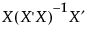• When formulas are created, they are entered as Formula column properties.
 • For many of the new columns, a Notes column property is added describing the column and indicating that Fit Least Squares created it.
Creates a new column called Pred Formula <colname> that contains both the formula and the predicted values. A Predicting column property is added, noting the source of the prediction.
Note: Pred Formula <colname> inherits certain properties from <colname>. These include Response Limits, Spec Limits, and Control Limits. If you change these properties for <colname> after saving Pred Formula <colname>, they will not update in Pred Formula <colname>.
See Prediction Formula for more details.
Creates a new column called Predicted <colname> that contains the predicted values computed by the specified model. Both a Notes and a Predicting column property are added, noting the source of the prediction.
Note: Predicted <colname> inherits certain properties from <colname>. These include Response Limits, Spec Limits, and Control Limits. If you change these properties for <colname> after saving Predicted <colname>, they will not update in Predicted <colname>.
Creates a new column called Residual <colname> that contains the observed response values minus their predicted values.
Creates two new columns called Lower 95% Mean <colname> and Upper 95% Mean <colname>. These columns contain the lower and upper 95% confidence limits for the mean response.
Creates two new columns called Lower 95% Indiv <colname> and Upper 95% Indiv <colname>. These columns contain lower and upper 95% confidence limits for individual response values.
Creates a new column called Studentized Resid <colname>, which contains the residuals divided by their standard errors.
Creates a new column called h <colname>. The column values are the diagonal values of the matrix, sometimes called hat values.
Creates a new column called StdErr Pred <colname> that contains the standard errors of the predicted mean response.
Creates a new column called StdErr Resid <colname> that contains the standard errors of the residual values.
Creates a new column called StdErr Indiv <colname> that contains the standard errors of the individual predicted response values.
 • X Leverage of X for R
 • Y Leverage of X for R
Creates a new column called Cook’s D Influence <colname>, which contains values of the Cook’s D influence statistic.
Creates a new column called PredSE <colname> that contains both the formula and the values for the standard error of the predicted values.
Creates two new columns called Lower 95% Mean <colname> and Upper 95% Mean <colname>. These columns contain both the formulas and the values for lower and upper 95% confidence limits for the mean response.
Creates two new columns called Lower 95% Indiv <colname> and Upper 95% Indiv <colname>. These columns contain both the formulas and the values for lower and upper 95% confidence limits for individual response values.
Pred Formula <colname> differs from Predicted <colname> in that it contains the prediction formula. Right-click in the Pred Formula <colname> column heading and select Formula to see the prediction formula. The prediction formula can require considerable space if the model is large. If you do not need the formula with the column of predicted values, use the Save Columns > Predicted Values option. For information about formulas, see the Using JMP book.
Note: If you select Graph > Profiler to access the profilers, first save the formula columns to the data table using Prediction Formula and StdErr Pred Formula. Then place both of these formulas into the Y, Prediction Formula role in the Profiler window. After you click OK, specify whether you want to use PredSE <colname> to construct confidence intervals for Pred Formula <colname>. Otherwise, JMP creates a separate profiler plot for PredSE <colname>.